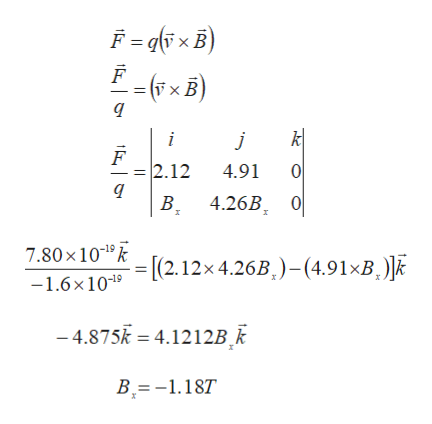# An electron moves through a uniform magnetic field given by B vector= Bx i+(4.26 Bx)j. At a particular instant, the electron has velocity v vector = (2.12i+4.91j) m/s and the magnetic force acting on it is (7.80 × 10-19 )k N. Find Bx.

Question
255 views

An electron moves through a uniform magnetic field given by B vector= Bx i+(4.26 Bx)j. At a particular instant, the electron has velocity v vector = (2.12i+4.91j) m/s and the magnetic force acting on it is (7.80 × 10-19 )k N. Find Bx.

check_circle

Step 1

Given

The magnetic field B = Bx i + (4.26 Bx) j

The velocity of the electron v = (2.12 i + 4.91 j) m/s

The force acting on the electron F = (7.80 x 10-19 k ) N

Known

The magnitude of the charge of electron q = e = 1.6 x 10-19 C

Step 2

Understanding

The electron moves in the xy plane

The magnetic field is acting in the xy plane

The force is acting in the positive z axis direction

Formula Used

When a charges particle is moving in a magnetic field, the charged particle will experience a force in the direction perpendicular to both the velocity and the magnetic field. This force is called magnetic Lorentz force

Step 3

Solution

...help_outlineImage TranscriptioncloseF -xB) F fF B) i j 2.12 4.91 0 4.26B 0 B 7.80 x 1019 k [(2.12x 4.26B.)-(4.91*B -1.6x109 -4.875 4.1212B_k B-.187 fullscreen

### Want to see the full answer?

See Solution

#### Want to see this answer and more?

Solutions are written by subject experts who are available 24/7. Questions are typically answered within 1 hour.*

See Solution
*Response times may vary by subject and question.
Tagged in

### Magnetism